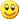• spmd的思想则是不同的数据，用同一个程序处理。当然这个程序内部可以编写针对不同情况的处理代码。spmd的内部实现代码限制很少，spmd的灵活性比parfor要高得多。spmd的使用方法如下：startmatlabpool(2)spmda = ...
parfor的并行思想是把同一批数据分给for循环中不同的循环体，进行处理。spmd的思想则是不同的数据，用同一个程序处理。当然这个程序内部可以编写针对不同情况的处理代码。spmd的内部实现代码限制很少，spmd的灵活性比parfor要高得多。spmd的使用方法如下：startmatlabpool(2)spmda = magic(labindex )%代码endclosematlabpool;结果：Lab 1:a =1Lab 2:a =1     34     2为了处理不同的情况，spmd实际运行时会获取当前线程编号，用常量labindex表示，等同于openMP的omp_get_thread_num()。问题一：如何在外部手动分配数据？startmatlabpool(2)p = Composite();p{1} = rand(1,3);p{2} = 'hello';spmddisp(p);endclosematlabpool;结果：Lab 1:0.793866004096169         0.536373818464747         0.647201517107599Lab 2:hellocomposite数据是spmd并行结构中最简单的数据，每个labindex线程只访问composite{labindex}数据。不可越界。在关闭并行环境后所有的composite数据都没了。composite数据每个部分可以有不同的数据类型。问题二：分布式计算可以用spmd吗？of course。不过我也没有多台可以分布式计算的电脑，只能试验一下了。分布式计算的一大问题是数据的管理。下面分为两个部分：1、客户端分配客户端(client)使用distributed语句产生分布式数据。默认根据最后一个维度来分配数据，你也可以自己调。下面源码：startmatlabpool(2)a = rand(5,2);p1 = Composite();b = rand(5,2);for i = 1:2p1{i} = a(:,i);endp2 = distributed(b);%p2 = distributed.rand(5,2);%同样可以这样干spmddisp([p1 getLocalPart(p2)]);endclosematlabpool;结果：Lab 1:0.101441167942127         0.8853259311029330.395333513944682         0.1405057593001160.604619875183008        0.06798444413856490.102777340759248         0.8909171468775930.603123131497137         0.862605458737845Lab 2:0.68058153278091         0.2395783847671410.895975215333032         0.4359247608324130.584621985559958         0.8318290007151150.972073878349711         0.2128873277462070.872288140538251          0.18323345771615请注意获取p2要使用getLocalPart语句。2、服务端(线程计算端)分配startmatlabpool(2)spmdX = [1 2;4 5];codist = codistributor1d(1, [1 1]);%1表示第一维度切割，行切割，[1 1]表示每个lab分配一行数据C = codistributed(X, codist);disp([labindex, numlabs]);disp(getLocalPart(C));endclosematlabpool;结果Lab 1:1     21     2Lab 2:2     24     5
展开全文• 三天前拿到网购的《实战matlab并行》一书，由于时间紧迫，大概拜读了一遍，但还有还多问题没弄明白，主要问题是：parfor和spmd这两种并行结构能否嵌套使用？如能，请举例说明？1、我的程序介绍：% Spp3=zeros(nwinds...
三天前拿到网购的《实战matlab并行》一书，由于时间紧迫，大概拜读了一遍，但还有还多问题没弄明白，主要问题是：parfor和spmd这两种并行结构能否嵌套使用？如能，请举例说明？1、我的程序介绍：%      Spp3=zeros(nwinds,nwinds,nf);%      for i=0:duanshu-1                          %duanshu是按重叠率50%划分的段数%          Pt=Fluc(:,512*i+1:512*(i+2)).*window2;   %乘以窗函数 nfft/2=512;维数：216*1024%          pfT=fft(Pt,nfft,2);   %对外荷载时程进行傅里叶变换,对每列进行变换，(216*1024); pfT1=conj((fft(Pt)')');%          for j=1:nf    % 可取nf(513，半谱)，也可取1024(全谱)%              Sp3(:,:,j)=conj(pfT(:,j))*(pfT(:,j).') ; % 激励谱矩阵，考虑了交叉谱的作用；%          end%          Spp3=Spp3+Sp3;    %将各段的位移自功率谱叠加%      end%      MC=duanshu*Fs*nfft*K0;    % Modifying Coeffcient,namely:Spp(i,/duanshu/T/Fs/Fs/K0;%      Spp3=Spp3/MC;             % 对激励谱矩阵修正；%      clear Sp3;% % %      Syy=zeros(DOF,nf);      % 前面已有，故此不重复；%      for j=1:nf%         hp=diag(H(:,j));  %  向量 变为 对角阵；文献中hp(f)%         spp=Spp3(:,:,j); % 216*216%         HH=Fi*hp*Fi'*R;%         Syy(:,j)=abs(diag(conj(HH)*spp*HH.'));%         %------应力谱---------------%%         Hr=ModalStr*hp*Fi'*R;%         Srr(:,j)=abs(diag(conj(Hr)*spp*Hr.'));%      end2、改为并行结构%% 并行计算修改--完全二次型组合法(CQC)-----------------------------%Spp3=zeros(nwinds,nwinds,nf);Sp3=zeros(nwinds,nwinds,nf);spmd,                                         % 10 labs needed；相同程序，不同数据；pts=0:duanshu-1   ,                       % duanshu是按重叠率50%划分的段数i=pts(labindex),                          % 以下程序任务由编号为labindex的worker做；P_temp=Fluc(:,512*i+1: 512*(i+2));codist=codistributor1d(1,[ ]);co_P=codistributed( P_temp ,codist);cpLocal=getLocalPart(co_P);Pt=cpLocal.*window2;   %乘以窗函数 nfft/2=512;维数：216*1024pfT=fft(Pt,nfft);      %对外荷载时程进行傅里叶变换,(每个worker处理一个列向量的fft变换)%          Pt=P_temp.*window2;   %乘以窗函数 nfft/2=512;维数：216*1024%          pfT=fft(Pt,nfft,2);   %对外荷载时程进行傅里叶变换,默认对每列进行变换，现转为对行进行变换，(216*1024); pfT1=conj((fft(Pt)')');for j=1:nf    % 可取nf(513，半谱)，也可取1024(全谱)Sp3(:,:,j)=conj(pfT(:,j))*(pfT(:,j).') ; % 激励谱矩阵，考虑了交叉谱的作用；endSpp3=Spp3+Sp3;    %将各段的位移自功率谱叠加endMC=duanshu*Fs*nfft*K0;    % Modifying Coeffcient,namely:Spp(i,/duanshu/T/Fs/Fs/K0;Spp3=Spp3/MC;             % 对激励谱矩阵修正；clear Sp3;% %      Syy=zeros(DOF,nf);      % 前面已有，故此不重复；parfor j=1:nfhp=diag(H(:,j));  %  向量 变为 对角阵；文献中hp(f)spp=Spp3(:,:,j); % 216*216temp=hp*Fi'*R;HH=Fi*temp;Syy(:,j)=abs(diag(conj(HH)*spp*HH.'));%------应力谱---------------%Hr=ModalStr*temp;Srr(:,j)=abs(diag(conj(Hr)*spp*Hr.'));end3、问题：这一段程序是parfor循环体中的一个子程序中的一段，想请问spmd能否在parfor循环中嵌套使用，若可以请给出修改建议，拜谢！
展开全文• 1. matlab仿真模型怎么并行计算以单台双核计算机为例。首先打开MATLAB命令窗口，输入...主要是两种：parfor模式和spmd模式。两种模式的应用都很简单。第一个中，parfor其实就是parallel+for简化而来，顾名思义啊，...
1.    matlab仿真模型怎么并行计算以单台双核计算机为例。首先打开MATLAB命令窗口，输入matlabpool open就OK了。这样，就相当于将一台计算机的两个核心，当做两台机器用啦。接下来是编程序实现的方法。MATLAB并行计算的模式有几种？主要是两种：parfor模式和spmd模式。两种模式的应用都很简单。第一个中，parfor其实就是parallel+for简化而来，顾名思义啊，就是把原来程序中的for循环中的关键字forg改为parfor就OK啦。不过这是很初步的介绍，限于篇幅不能详细展开了，详细的可以用输入命令help parfor查看：同样地，可以适用于spmd模式。程序举例：例如很简单的一个程序：tic%传统方式计算c1=1;for i = 1:500c1 = c1+max(eig(rand(i,i)));endt1 = toc;matlabpool open;%parfor并行方式计算ticc2=1;parfor ii = 1:500c2 = c2+max(eig(rand(ii,ii)));endt2 = toc;matlabpool close;display(strcat('parfor并行计算时间：'，num2str(t2)，'秒'))；display(strcat('客户端串行计算时间：'，num2str(t1)，'秒'))；分别用传统方式算和parfor算，时间区别如下图所示：这只是用双核计算的效果，如果是4核心8核心，效果要好的多啦，需要用MATLAB的童鞋不妨试试看咯。关闭并行计算模式。2.    怎么处理matlab并行计Matlab并行运算目前，新购置的电脑大部分都是多核的了，使用Matlab进行大量计算时如何有效利用多核呢？Matlab目前版本已经比较好的支持多核并行运算了。是用的Matlab版本是R2007b。电脑是双核的。先简单试试：>> matlabpool local 2Submitted parallel job to the scheduler, waiting for it to start.Connected to a matlabpool session with 2 labs.显示正在进行多核配置，然后，提示连接到2个“实验室”(labs)。我这也理解的：本地虚拟出2台可以运行matlab的工作站，这样用分布式计算工具箱可以进行并行计算(matlabpool这个命令好像是在并行计算工具箱里的)。>> testParallelElapsed time is 7.750534 seconds.这里运行testParallel函数，已经开辟了2个labs，为了进行多核并行运算，testParallel中，要用parfor代替原来的for循环。在运行这个时，观察windows任务管理器，可以发现一共有3个MATLAB.exe进程。其中一个占内存较多的，应该是主控的，他基本不干活，只负责分配，进行计算时他的cpu占用率只有1~2%，剩下两个进程专门用来计算的，跑起来各占cpu 49%左右。看上去还是每个matlab进程单核运算，但是一下开2个进程，所以能把cpu用满。当运行完testParallel后，三个进程的cpu都立刻降为1%左右了。3.    matlab仿真模型怎么并行计算以单台双核计算机为例。首先打开MATLAB命令窗口，输入matlabpool open就OK了。这样，就相当于将一台计算机的两个核心，当做两台机器用啦。接下来是编程序实现的方法。MATLAB并行计算的模式有几种？主要是两种：parfor模式和spmd模式。两种模式的应用都很简单。第一个中，parfor其实就是parallel+for简化而来，顾名思义啊，就是把原来程序中的for循环中的关键字forg改为parfor就OK啦。不过这是很初步的介绍，限于篇幅不能详细展开了，详细的可以用输入命令help parfor查看：同样地，可以适用于spmd模式。程序举例：例如很简单的一个程序：tic%传统方式计算c1=1;for i = 1:500c1 = c1+max(eig(rand(i,i)));endt1 = toc;matlabpool open;%parfor并行方式计算ticc2=1;parfor ii = 1:500c2 = c2+max(eig(rand(ii,ii)));endt2 = toc;matlabpool close;display(strcat('parfor并行计算时间：'，num2str(t2)，'秒'))；display(strcat('客户端串行计算时间：'，num2str(t1)，'秒'))；分别用传统方式算和parfor算，时间区别如下图所示：这只是用双核计算的效果，如果是4核心8核心，效果要好的多啦，需要用MATLAB的童鞋不妨试试看咯。关闭并行计算模式。4.    matlab并行计算需要设置吗需要的方法一、图形界面设置如图所示位置，单击，选start parallel poolcommand window中会提示Starting parallel pool (parpool) using the 'local' profile 。当并行工具箱启动后会提示链接到本地*个核心。方法二、command启动并行CoreNum=2； %设定机器CPU核心数量if matlabpool('size')<=0 %判断并行计算环境是否已然启动matlabpool('open','local',CoreNum)； %若尚未启动，则启动并行环境elsedisp('Already initialized')； %说明并行环境已经启动。end。5.    matlab中如何使用并行运算进行多行语句同时运行可以尝试用 parfor 写入循环，也可以用 batch 命令进行批处理：j = batch(fcn,N,{x1, 。, xn});wait(j) % Wait for the job to finishdiary(j) % Display the diaryr = fetchOutputs(j); % Get results into a cellarrayr{1} % Display resul具体操作可以参考帮助文档。像这样的问题其实不用并行计算也可以的，有其它方法也可以加快速度，比如使用bsxfun函数，可以同时计算A,B,C中元素：C = bsxfun(fun, A, B)像题主的程序中有四个同样的运算，就可以这样写：C = bsxfun(SRD, SN,GD,DN,RN)其中 C 包含了四个元素，SN,GD,DN,RN分别为函数SDR 输入的第一、二、三、四个参数矩阵(向量)6.    matlab并行计算需要设置吗需要的方法一、图形界面设置如图所示位置，单击，选start parallel poolcommand window中会提示Starting parallel pool (parpool) using the 'local' profile 。当并行工具箱启动后会提示链接到本地*个核心。方法二、command启动并行CoreNum=2； %设定机器CPU核心数量if matlabpool('size')<=0 %判断并行计算环境是否已然启动matlabpool('open','local',CoreNum)； %若尚未启动，则启动并行环境elsedisp('Already initialized')； %说明并行环境已经启动。end
展开全文• end end % 用parfor和spmd命令都会使函数文件的global 命令失效 a和b=[]; % 答复：您忘了初始化吧，global在matlab中应该不受影响，见下例。 >>global g_data >>g_data = 10; >>mytestglobal ans = 55 function ...
% 问题1：我的计算程序数据比较繁杂 所以我在脚本文件中把很多变量设为全局变量 调用函数的时候就很方便% 问题简述如下:% 脚本文件global a ba=rand(3);b=rand(3);c=magic(3);d=rand(3);out1=func(c)out2=func(d)% 函数文件function out=func(c)global a bout=a+b+c;% 并行计算的脚本文件global a ba=rand(3);b=rand(3);c=magic(3);d=rand(3);spmdif labindex==1out1=func(c);elseif labindex==2out2=func(d);endend% 用parfor和spmd命令都会使函数文件的global 命令失效 a和b=[];%答复：您忘了初始化吧，global在matlab中应该不受影响，见下例。>>global g_data>>g_data = 10;>>mytestglobalans =55function [out] = mytestglobal()%function [out] = mytestglobal()global g_data;if isempty(g_data)g_data = 100;%     return;endout = 0;parfor kk = 1:g_dataout = out + kk;end建议：最好不用global，这种程序最不好管理，容易出问题。直接作为参数输入给matlab即可。现在matlab函数对阵列应该是地址传递，全局变量没有什么优势。% 问题2：在用spmd命令的时候不知道如何获取计算结果n1=8a=rand(n1,n1);n=matlabpool('size');%n=4  4labsspmdcodist=codistributor1d(1,ones(1,n)*n1/n,[n1 n1]);b=codistributed(a,codist);c=func(b);endgather(c);d=a-c;% 如果我的函数文件如下：function c=func(b)c=b;% 这个程序计算结果是没有问题的，但是我如果用循环就总是报错function c=func(b)[e f]=globalIndices(b,2);[g h]=globalIndices(b,1);c=zeros(h-g+1,f-e+1);for i=e:ffor j=g:hc(i,j)=b(i,j);endend答复如下，将上述实例修改为n1=8a=rand(n1,n1);n=matlabpool('size');%n=4  4labsspmdcodist=codistributor1d(1,ones(1,n)*n1/n,[n1 n1]);b=codistributed(a,codist);c=func(b);endfunction c=func(b)c = getLocalPart(b);注意：c此时为Composite对象，调用和查看方式为c{1}和c{2}class(c)ans =Compositec{1}ans =0.3643    0.5870    0.1958    0.0732    0.7637    0.6789    0.7566    0.58020.5323    0.4139    0.9924    0.5910    0.5588    0.4035    0.9955    0.53100.7117    0.3091    0.8023    0.9102    0.1838    0.9350    0.9624    0.90120.8715    0.2638    0.4242    0.1938    0.4979    0.4795    0.5351    0.5406% 求大师讲解！！
展开全文• matlab中使用matconvnet进行多线程、多GPU训练任务时，若出现： 1） warning: The SPMD infrastructure has been initializing for xx seconds. This may indicate a problem in initialization. You might ...
• pool = parpool('local',4); p=gcp('nocreate');...spmd A = rand(3,2); %generate a matrix A for each lab(worker) end for i = 1:length(A) figure; imagesc(A{i}); end delete(pool);
• matlab 其实可以在多cpu做并行计算，其中一个模式是 spmd 在命令行输入（matlab r2014a）： parpool 然后可以用spmd模式进行并行计算。 用法如下： spmd statements end 其中statements 为需要并行运行...高性能计算 并行计算
• spmd的思想则是不同的数据，用同一个程序处理。当然这个程序内部可以编写针对不同情况的处理代码。spmd的内部实现代码限制很少，spmd的灵活性比parfor要高得多。 spmd的使用方法如下： startmatlabpool(2) spmd a ...并行计算
• 从官方网站找到了解决方法 ...在matlab命令行中输入： license checkout Distrib_Computing_Toolbox 如果ANS=1，则进行下一步排查，否则可以激活一下证书再看看。 关闭local mpiexec功能 distcomp.feature( 'Local...
• parfor循环或spmd块的...因此，MATLAB®在运行时出现错误。 X = 5; parfor ii = 1:4 eval('X'); end X = 5; spmd eval('X'); end 类似地，您不能通过在parfor或spmd语句中执行clear来清除工作空间中的变量: parf
• SPMD（Single Program/Multiple Data）单程序多任务进行任务并行： ...你在一个Matlab客户端上运行一个程序，被标志为spmd模块的其他部分运行在其他lab上。当这些块运行完毕后，你的程序继续在客户端运
• ## Matlab并行编程方法

万次阅读 多人点赞 2014-01-03 08:08:27
本文讲一下matlab中的并行方法与技巧。分为以下几个板块： 1. 什么东西好并行？ 2. 怎么并行？ 3. parfor vs. SPMD 4. 注意事项及经验总结
• matlab开发-WorkerObjectWrapper。简化Parfor循环和SPMD块中的大型数据等资源管理控制系统
• ## Matlab并行编程

千次阅读 2017-04-27 19:57:33
本文讲的Matlab并行编程包括并行for循环和数据并行（同一个程序运行在不同的数据上），分别对应于parfor、spmd方法 1. 并行方法 parpool %开启并行池（parallel pool） %parallel program delete(gcp('nocreate')...并行编程
• 第3章为SPMD并行结构；第4章为其他Matlab并行结构；第5章为Matlab并行计算数据类型；第6章为Matlab通用并行程序设计；第7章为MDCE配置；第8章为创建多线程MEX文件；第9章为在Matlab中应用OpenMP进行并行计算；第10章
• 主要是想在server上实现 matlab 的 并行运算，问了一些人，大概有两个思路。（p.s. server上是 linux 系统） ...另一种是MATLAB的并行，用spmd或parfor，根据具体代码修改。" 最首先是关于 matlab parall
• 本文讲一下matlab中的并行方法与技巧，这里我们不涉及GPU加速，主要考虑for循环并行和数据并行。分为以下几个板块：1. 怎么并行？2. parfor vs. SPMD3. 注意事项及经验总结----------------------------------------...
• 转自Mathworks官方的视频教程，原教程标题为：Parallel and GPU Computing Tutorials一共有9个视频教程，由于公众号文章一次只能发3个视频，我们就分3次来发Matlab并行计算 GPU加速 视频教程Part1~3Matlab并行计算 ...
• p=parpool NumWorkers代表worker的个数，这里开启了...“SpmdEnabled”指定池是否可以运行 SPMD 代码 single-program-multiple-data(SPMD) 最常见的并行技术风格 %串行 for i=1:1024 A(i) = sin(i*2*pi/1024); e...
• 1. 首先打开并行运算： 在命令窗口输入：matlabpool open， 2013rb以后的MATLAB版本，matlabpool open 改成parpool 2. 主要是两种：parfor模式和spmd模式。两种模式的应用都很简单。第一个中，parfor其实就是...
• 第3章为SPMD并行结构；第4章为其他Matlab并行结构；第5章为Matlab并行计算数据类型；第6章为Matlab通用并行程序设计；第7章为MDCE配置；第8章为创建多线程MEX文件；第9章为在Matlab中应用OpenMP进行并行计算；第10...
• MATLAB并行运算的基本操作： 并行计算-并行工具箱 parfor spmd 分布式计算-分布式计算工具箱 mdcs配置# matlabspmdmatlab 订阅